# Catch up on the latest AI articles# Designing Proteins With Machine Learning! [Fold2Seq]

3 main points
✔️ Representation of protein 3D structure as the density of secondary structure in a finely divided unit cube, with the representation of each 3D structure acquired from a Transformer Encoder-based deep learning model
✔️ By learning embeddings that represent both the 3D structure and amino acid sequence of a protein, we captured the relationship between the different domains of 3D structure space and sequence space.
✔️ Outperformed RosettaDesign, SOTA, in terms of perplexity and sequence recovery rate in benchmarks

The images used in this article are from the paper, the introductory slides, or were created based on them.

## first of all

Proteins are made up of linear chains of amino acids and are one of the most important elements that form our bodies. Proteins do not have a linear structure but are folded into a desired three-dimensional structure to perform their biological functions. This folding of proteins is called folding.

Recently, the problem of designing an amino acid sequence to give a specific structure has attracted much attention, and this problem is called Inverse protein design. This problem is called "Inverse protein design". The challenges in this problem are " the vastness of the sequence space to be explored " and "the difficulty of mapping between the structure space and the sequence space ".

Most of the conventional studies on inverse protein design are based on the main chain structure of the protein, and few studies are based on the protein fold. However, it has been pointed out that it is difficult to design brand-new sequences and the diversity of the designed sequences is impaired in the sequence design by giving the protein main chain structure. This is because the fold is a higher-order representation than the main chain structure, and limiting the main chain structure implicitly narrows the candidate amino acid sequences.

Therefore, we try to design sequences by giving folds instead of main-chain structures in the paper presented here. Our main goals are to obtain a representation that guarantees the diversity of protein folds and to overcome the complex relationship between fold space and sequence space.

## Fold2Seq

### How to represent protein folds

Fold in proteins is a three-dimensional arrangement of local secondary structure elements (SSEs). The authors divide the three-dimensional space occupied by the protein structure into unit cubes and use the density of secondary structure elements (SSEs) in each cube as a representation of the fold.

There are four types of secondary structure elements considered: helix, β-strand, loop, and bend-turn. The influence of a certain amino acid residue $j$ of the protein on the unit cube $i$ is represented by a Gaussian distribution and multiplied by the corresponding One-Hot representation $t_j$ for each secondary structure element to obtain the following feature vector.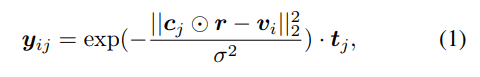By adding these feature vectors together concerning amino acid residues, the density of protein secondary structure in a certain cube can be represented as a mixed Gaussian distribution derived from each amino acid residue.

The image of the calculation of the fold representation is shown in the following figure.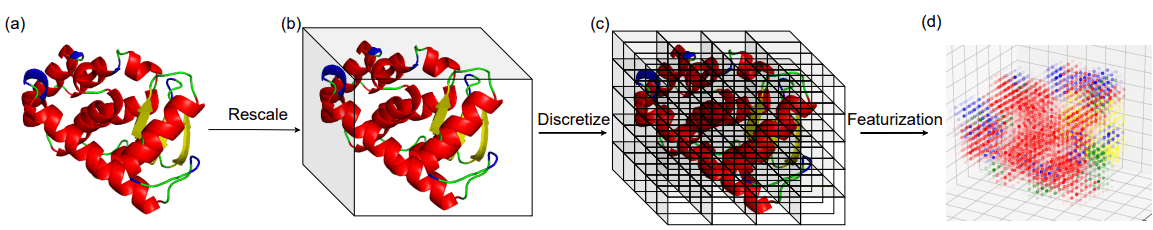### Architecture and loss functions

The relationship between the Fold2Seq architecture and the loss function is shown below.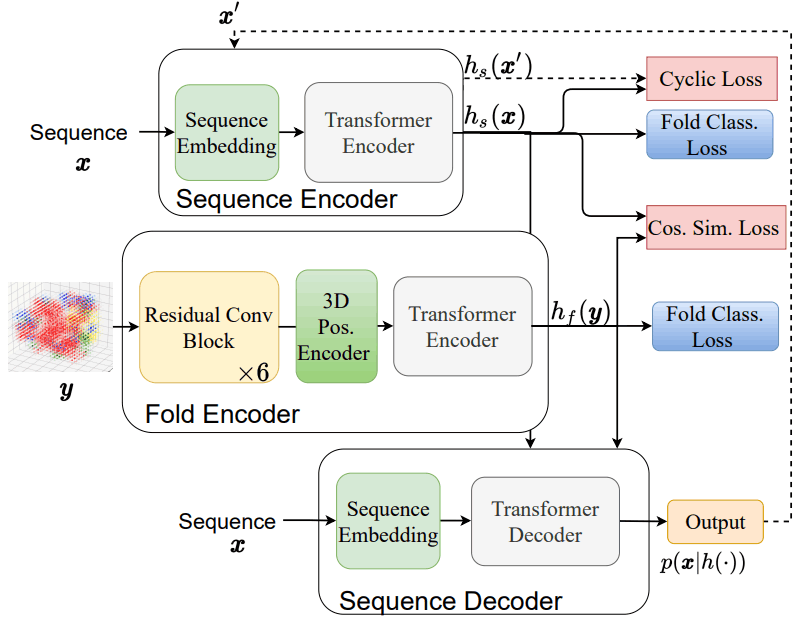This architecture is made up of the following three elements

1. Array Encoder $h_s$: It is responsible for dropping the amino acid sequence into the latent space, and employs the conventional Transformer Encoder.
2. Fold encoder $h_f$: it is responsible for dropping the protein fold into the latent space, and it employs a residual neural network composed of 3D convolutional layers.
3. Array Decoder $p(x|h(.))$: This is responsible for outputting amino acid sequences from latent space, and is a conventional Transformer Decoder.

Fold2Seq training incorporates the framework of Joint Embedding Learning, which states that two intra-domain losses and one cross-domain loss are required to successfully capture relationships between distant domains such as 3D structures and sequences. domain) is necessary to successfully capture the relationships between distant domains such as 3D structures and sequences.

Intra-domain loss is the loss of placing sequences or folds that have the same function in vivo close to each other in latent space, and inter-domain loss is the loss of placing sequences and folds that have the same function in vivo close to each other in latent space.

The overall loss for Fold2Seq is shown below. We will look at each term in more detail below.

####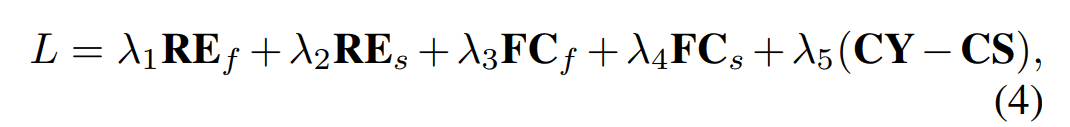$RE_f, RE_s$.

This is a simple reconstruction loss in the autoencoder model. We distinguish the loss of reconstructing an array with input folds as $RE_f$ and the loss of reconstructing an input array as $RE_s$.

#### $FC_f, FC_s$.

This loss corresponds to the intra-domain loss described above. We classify the class label of each protein fold from the feature vector obtained by averaging the output $h_s(x)$ of the array encoder and $h_f(y)$ of the fold encoder in the length direction, respectively. We define the cross-entropy loss in this task for the sequence and the fold respectively as $FC_s, FC_f$.

By including this classification task, sequences and folds belonging to the same class become similar latent representations and thus also serve as interdomain losses.

#### $CS$.

Cosine similarity is calculated for the output $h_s(x)$ of the array encoder and $h_f(y)$ of the fold encoder. The effect is to make the latent representation close between the corresponding fold and array sites.

#### $CY$.

This section is based on the cyclic loss of CycleGAN. The array generated by the fold encoder and the array decoder is input to the array encoder to obtain the latent representation $h_s(x')$. Then we restrict the generated sequence not to be far from the original sequence by setting the L2 distance from the latent representation $h_s(x)$ of the original sequence as the loss.The network architecture and loss functions are illustrated below, and the model training and sequence generation is shown in Figure 1.

When training a model using the loss function described above, if the array encoder and the fold encoder are trained at the same time, the training of the fold encoder does not progress, so the authors propose a two-step training method as follows.

1. $L_1=\lambda_2 RE_s + \lambda_4 FC_s$ to learn array encoder and array decoder
2. Fix the weights of the array encoder and learn the fold encoder and array decoder by $L_2=\lambda_1 RE_f + \lambda_3 FC_f + \lambda_5 (CY - CS)$.

After training the model, the actual generation of sequences from protein folds is done by conventional autoregressive inference with Transformer. The procedure for generating sequences is shown in the figure below.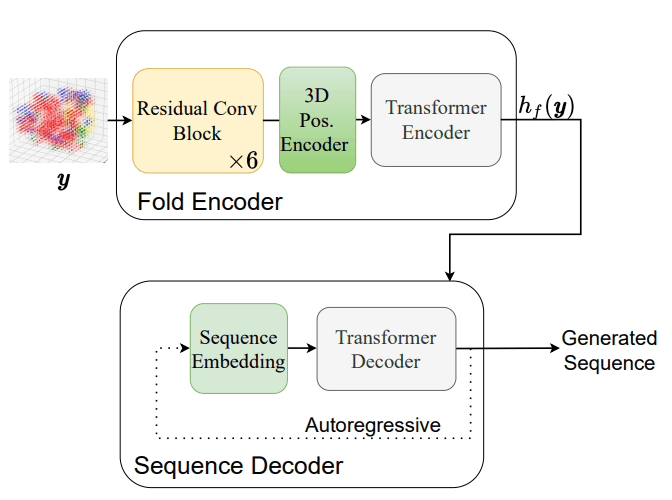## Evaluation of the designed array

To evaluate the quality of sequences generated using Fold2Seq, we have defined four structural-level evaluation metrics. In this article, we will focus on two of them.

In the original paper, we used not only evaluation metrics on the sequence domain as shown below, but also evaluation metrics on the structure domain. Please refer to it if you are interested.

### Perplexity per amino acid residue

In the set of structures that make up the protein fold $i$, $S_i$, the perplexity is calculated for the sequences that belong to each structure. The value of the perplexity calculated for a fold $i$ is defined as follows. The smaller the value, the better the index.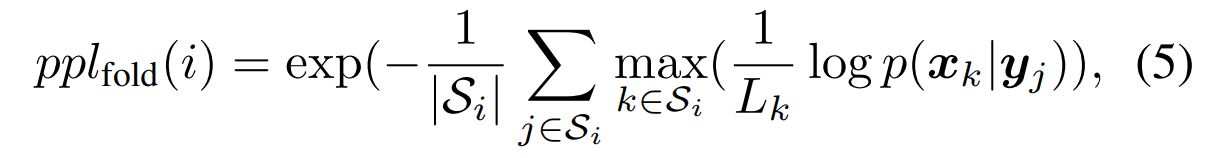### coverage

This is an index that selects one structure as a representative example from the fold $i$ and evaluates how much of the sequences belonging to the original fold $i$ could be generated by the sequence generated from that structure. The criterion for judging whether a sequence has been properly generated is a sequence similarity of 30% or more.

The specific calculation method is as follows. Let $G_k$ be the set of sequences generated from some structure k.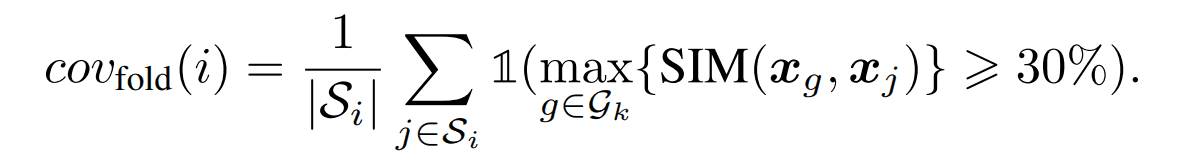## result

We briefly present the training results and evaluate Fold2Seq concerning CATH4.2, a benchmark that includes protein conformation and sequence information.

The table below shows the results of the evaluation of the perplexity of each amino acid residue. As a reference, the perplexity calculated by sampling amino acids from a uniform distribution (uniform) and the perplexity calculated by using the entire amino acid sequence of UniRef50 (natural) is shown.

In addition, cVAE and gcWGAN are comparison methods to design amino acid sequences from protein folds using a deep generative model, and Graph_trans is a comparison method to design sequences by inputting the main chain structure as a graph structure into the model.

We compare the performance on the test data set with overlap between training data and structure (ID Test) and the test data set without overlap (OD Test). The overall trend is that the OD Test has higher perplexity.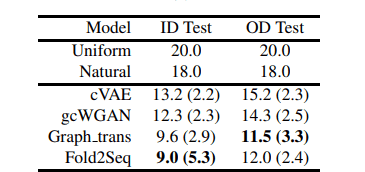From the above results, we can see that Fold2Seq outperforms other methods using deep generative models, and performs as well as when given high-resolution structural information such as the main chain structure.

The table below also shows the results of the coverage evaluation. We divided the folds in the test dataset according to the number of sequences belonging to the fold (3 or more) and calculated the coverage for each.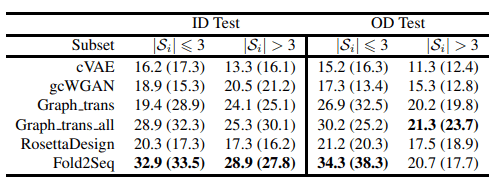From the results above, we can see that Fold2Seq better captures sequence diversity within the protein fold compared to existing methods, noting that it outperforms physics-based methods such as RossettaDesign.

The paper also argued for the superiority of Fold2Seq in terms of design time and robustness to the input of structures lacking amino acid residues and went on to discuss its practicality as a tool.

## summary

What did you think? When we think of machine learning, we have the impression that it has been developed in fields such as images and natural language, but it is surprising to see how machine learning has been applied to seemingly unrelated fields such as biology.

Recently, AlphaFold2, which predicts the three-dimensional structure of a protein from its sequence, has attracted a great deal of attention, and research in the opposite direction of predicting sequences from three-dimensional structures is likely to accelerate in the future.

It may not be long before we can design proteins that work as expected in vivo.

If you have any suggestions for improvement of the content of the article,